# 12022022 Radial acceleration AH Physics Radial acceleration Newtons

• Slides: 1212/02/2022 Radial acceleration AH Physics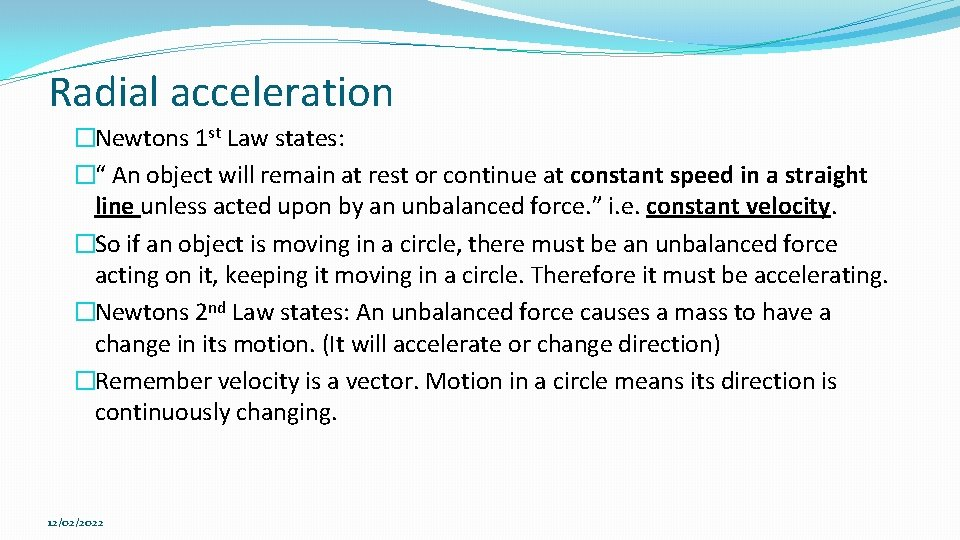Radial acceleration �Newtons 1 st Law states: �“ An object will remain at rest or continue at constant speed in a straight line unless acted upon by an unbalanced force. ” i. e. constant velocity. �So if an object is moving in a circle, there must be an unbalanced force acting on it, keeping it moving in a circle. Therefore it must be accelerating. �Newtons 2 nd Law states: An unbalanced force causes a mass to have a change in its motion. (It will accelerate or change direction) �Remember velocity is a vector. Motion in a circle means its direction is continuously changing. 12/02/2022Radial acceleration �How can an object traveling in a circle have a steady speed and be accelerating at the same time? �Well we’ve already met this when we studied satellite motion. A satellite has a constant tangential speed but it is continuously accelerating towards the Earth This acceleration is towards the centre of the circle (along the radius) and is called the radial acceleration 12/02/2022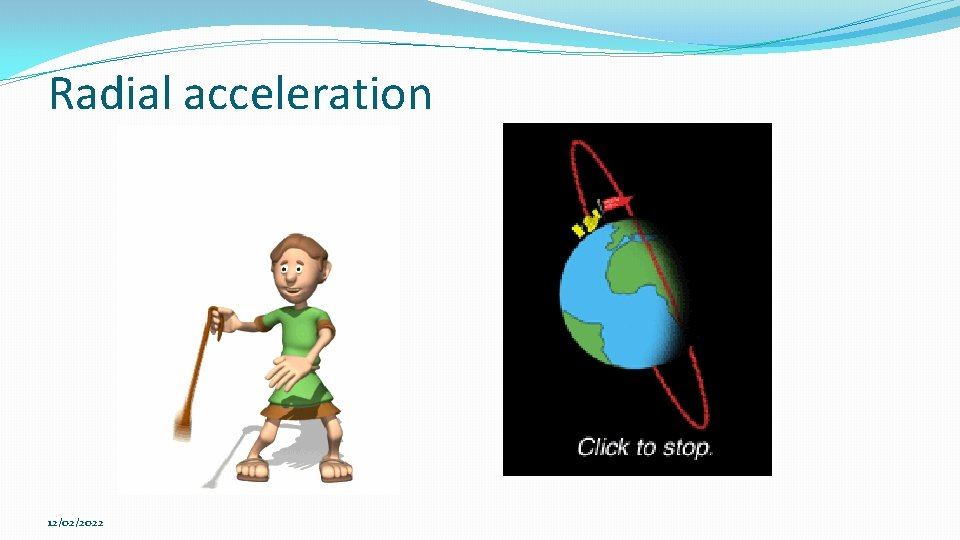Radial acceleration equation �What is the relationship between tangential speed (or angular velocity ) and radial acceleration ? 12/02/2022 You need to be able to derive this relationship… (unlikely to be in the exam)Radial acceleration derivation Consider a particle moving in a circle with a constant tangential speed. The instantaneous velocity is shown at 2 points: A and B. Initial speed is ‘u’ final speed is ‘v’ Velocity is a vector so the change in velocity (v-u) is found using the following vector diagram: 12/02/2022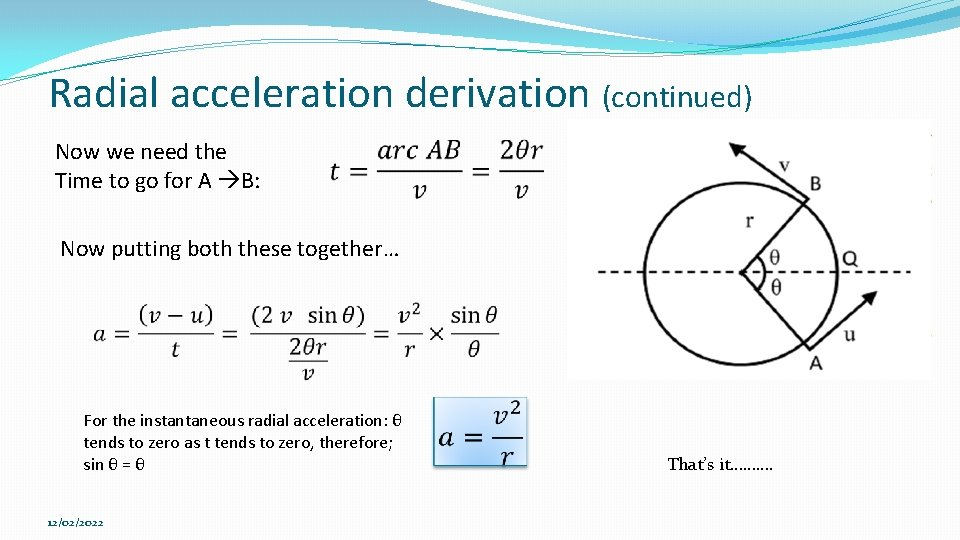Radial acceleration derivation (continued) Now we need the Time to go for A B: Now putting both these together… For the instantaneous radial acceleration: θ tends to zero as t tends to zero, therefore; sin θ = θ 12/02/2022 That’s it……….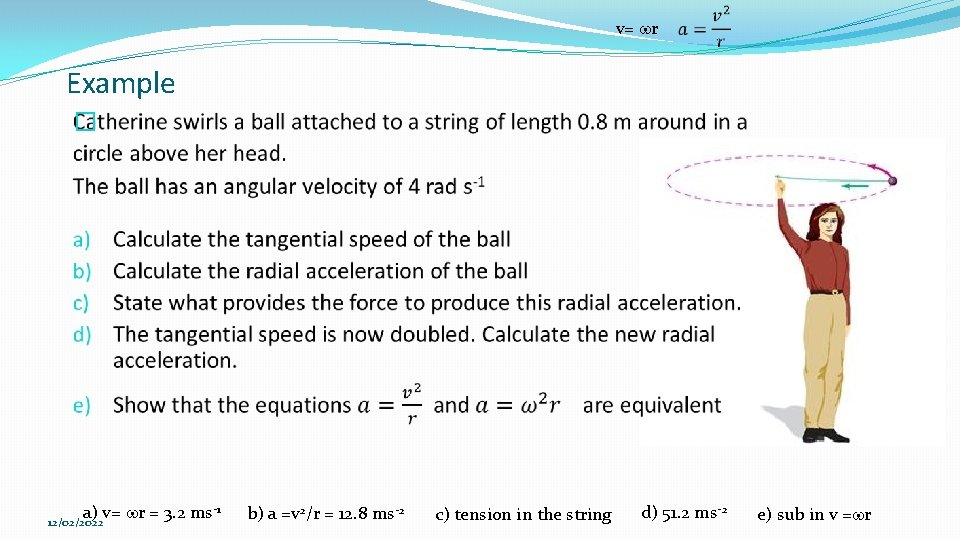v= wr Example � a) v= wr = 3. 2 ms-1 12/02/2022 b) a =v 2/r = 12. 8 ms-2 c) tension in the string d) 51. 2 ms-2 e) sub in v =wr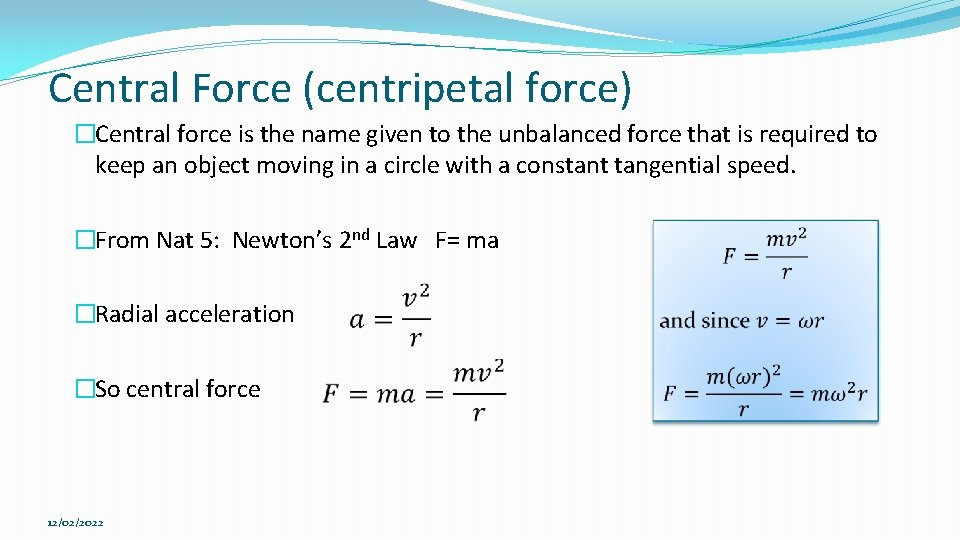Central Force (centripetal force) �Central force is the name given to the unbalanced force that is required to keep an object moving in a circle with a constant tangential speed. �From Nat 5: Newton’s 2 nd Law F= ma �Radial acceleration �So central force 12/02/202212/02/2022Centripetal v Centrifugal forces. �Centripetal force is the force required to keep an object moving in a circle. �Centrifugal force is a fictional force. It is not real. It is “what you feel” if you are moving in a circle. You feel as if you are being thrown out of the circle when in fact all your body is doing is trying to continue at a steady speed in a straight line (Newton’s 1 st Law) �What you feel is your reaction to the centripetal force keeping you moving in a circle. �Your being “thrown out” of a circular path is only you obeying Newton’s 1 st Law! 12/02/2022Structured Problems, page 8, Qs 36 -45. 12/02/2022